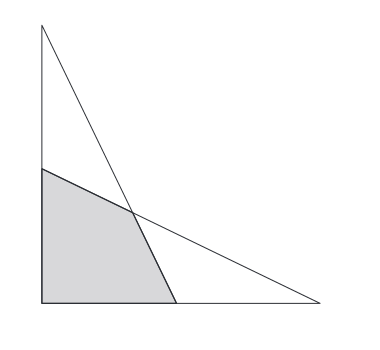# Area Without Numbers

Geometry Level 3Two right triangles, both with legs measuring $a$ and $b$, where $a>b$, are overlapping as the image above.

What is the area of the gray quadrilateral?

×